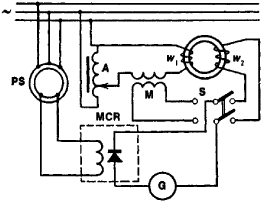# Ferrometer

## ferrometer

[fə′räm·əd·ər]
(engineering)
An instrument used to make permeability and hysteresis tests of iron and steel.
McGraw-Hill Dictionary of Scientific & Technical Terms, 6E, Copyright © 2003 by The McGraw-Hill Companies, Inc.
The following article is from The Great Soviet Encyclopedia (1979). It might be outdated or ideologically biased.

## Ferrometer

a device for determining the instantaneous values of the induction (Bt) and intensity (Ht) of the magnetic field in ferromagnetic specimens. A ferrometer makes it possible to plot, point by point, the symmetrical dynamic magnetic-reversal curves for ferromagnetic specimens (seeMAGNETIZATION CURVE) in magnetic fields that vary periodically (usually at the frequencies specified for mains used by industry) and to record magnetic-reversal curves on paper by means of a two-coordinate graphic recorder or on an oscilloscope screen.Figure 1. Electrical diagram of a ferrometer: (G) galvanometer, (MCR) mechanically controlled rectifier, (PS) phase shifter, (S) switch, (w1) magnetizing coil, (w2) measuring coil, (M) mutual-inductance coil, (A) autotransformer

Ferrometers operate on the principle by which the instantaneous values of Bt and Ht are proportional to the mean values of their derivatives over a specific time interval. The mean values of the varying electrical quantities in a ferrometer are measured by a galvanometer during a multiple half-period of the variation in the magnetic field. The galvanometer is equipped with a rectifier controlled by means of a phase shifter that determines the moment when the field begins acting on the ferrometer. The derivative of the induction dB/dt is obtained from the emf e in a measuring coil wound on the test specimen: e = –w2S(dB/dt), where w2 is the number of turns on the coil (Figure 1) and S is the cross section of the specimen. The instantaneous value of the magnetizing field intensity Ht is calculated from the value of the current i, for which the derivative is obtained from the value of the emf eM in the secondary winding of the mutual-inductance coil M (the primary winding w1, is connected in series with the magnetizing circuit): eM = –M(dl/dt), where M is the coefficient of mutual inductance of the coil.

In order to find the points of a dynamic magnetic-reversal curve, Bt, and Ht, are measured for several positions of the phase shifter (ordinarily for equal fractions of a period), and the curve is plotted from the data thus obtained. The primary magnetization curve is obtained from the locus of the symmetrical peaks on the dynamic magnetic-reversal curve.

In the USSR the model U-542 ferrometer is in series production; also manufactured are the high-frequency models F-2M and F-3 (up to 10 kilohertz).

### REFERENCES

Magnitnye izmereniia. Moscow, 1969.
Kifer, I. I. Ispytaniia ferromagnitnykh materialov, 3rd ed. Moscow, 1969. Page 197.

I. I. KIFER